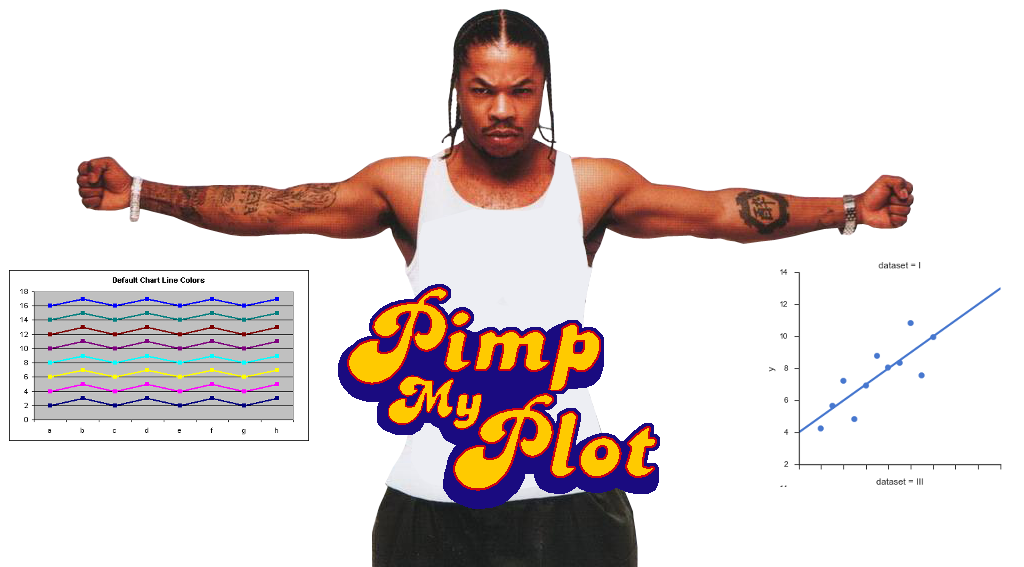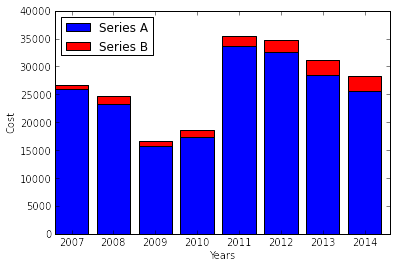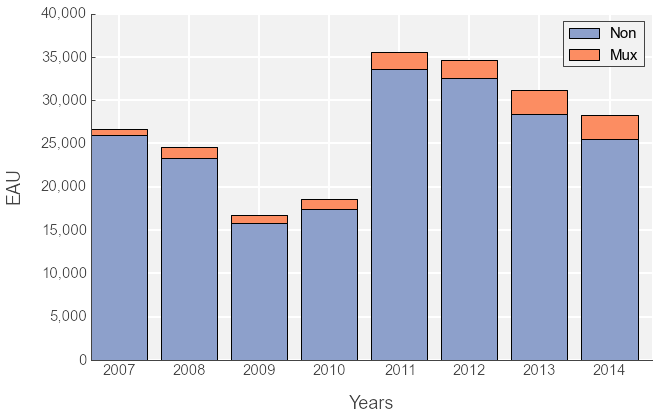# Pimp My Plots

October 28, 2014      Stardate: 68287.5     Tagged as: Python Matplotlib## Making Matplotlib Look Decent… aka Pimp my Plots¶

In this post I will show some matplotlib kung-fu! Most of this was stolen from @jakevdp, but others from StackExchange. The basic premise is that the default values for any visualization library (Excel, R, Python, Matlab) is ugly. Why do the developers use such horrible defaults?? But this tutorial, amongst many others on the interwebs, will show you how to “pimp my plots”!

In :
#I'll abstract out some of the mechanics to focus on the main idea of this post.
%run ~/Blog/draft/SWS/setup.py


Here I plot out some data that I developed in the file above. The contents are not important and are just used as an example to showcase the pplot formatting.

In :
%matplotlib inline
import matplotlib.pyplot as plt

plt.bar(range(0,8), non, color='b', label='Series A')
plt.bar(range(0,8), mux, color='r', bottom=non, label='Series B')
plt.xticks(np.arange(0.4,8.4,1), muxYear.index.values, rotation='horizontal')

plt.ylabel("Cost")
plt.xlabel("Years")
plt.legend(loc='best')

plt.show()#### Figure 1. “Before” Plot¶

The result is a pretty standard plot. The stacked bargraph shows two data series, A and B, across 7 years. The axes are labeled and everything looks like it is there. This isn’t a bad plot, I wouldn’t do much more than this if I were plotting a histogram or scatterplot to look at general data distribution. BUT, it isn’t “pretty” enough to present to management or a report or a journal. The main point is, if it’s a quick and dirty plot I don’t polish it, but if someone else will look at it, I make it optimal for the audience.

Who is your audience? Is this for a powerpoint slide? Then make sure all the text, ticks, etc. are large enough to be seen from the back of the room. Is this for an internal report? Then I crank up the resolution, throw in color, make it publication ready. Is this for a journal article? Then I consider doing all the plots in greyscale because it probably won’t be published in color.

OK… you weren’t reading this for a lecture. Let’s get to the fun stuff.

The key is changing your global rcParams to make the “default” better. Here is the guide to all matplotlib params. Also, I use the brewer2mpl library to ensure my colormaps are “correct”, this is optional but I recommend it. Either use the library or hardcode your colors from colorbrewer.

In :
# first import rcParams from matplotlib
from matplotlib import rcParams

# optional, use the colorbrewer color maps
import brewer2mpl

# my color map from colorbrewer, this is qualitative and colorblind safe
mycolors = brewer2mpl.get_map('Set2', 'Qualitative', 3).mpl_colors

# === Constants for figure size ===
# Springer book published chart width requirements (170 mm=~ 6.69291 inches)
WIDTH = 6.69291  # http://www.springeropen.com/authors/figures
# Golden ratio
PHI = 1.6180339887498948482  # https://en.wikipedia.org/wiki/Golden_ratio
HEIGHT = WIDTH / PHI

# === Figure ===
rcParams['figure.figsize'] = (WIDTH*1.5, HEIGHT*1.5)  # I like the image slightly bigger for online publishing
rcParams['figure.dpi'] = 400

# === Axes Style ===
rcParams['axes.labelsize'] = 18
rcParams['axes.titlesize'] = 22
rcParams['axes.labelweight'] = 'normal'
rcParams['axes.facecolor'] = 'f2f2f2'
rcParams['axes.edgecolor'] = '444444' # GREY
rcParams['axes.axisbelow'] = True
rcParams['axes.labelcolor'] = '444444' # GREY

# === Font Style ===
rcParams['font.size'] = 15
rcParams['font.family'] = 'Arial'

# === Legend Style ===
rcParams['legend.fontsize'] = 'medium'
rcParams['legend.frameon'] = True
rcParams['legend.numpoints'] = 3

# === Tick Style ===
rcParams['xtick.major.width'] = 1
rcParams['xtick.color'] = '444444' # GREY

rcParams['ytick.major.width'] = 1
rcParams['ytick.color'] = '444444' # GREY

rcParams['lines.linewidth'] = 2

# === Grid Style ===
rcParams['axes.grid'] = True
rcParams['grid.linestyle'] = '-'
rcParams['grid.alpha'] = 1
rcParams['grid.color'] = 'white'
rcParams['grid.linewidth'] = 2


The above code changes the global plot parameters, meaning anything you plot here on out will be affected. I like to put this in my path and import it when I plot, kinda like my own personal config file.

Below I plot out the same data as before, but with some additional “local” formatting.

In :
import matplotlib.ticker as tkr

# formatter function takes tick label and tick position
def func(x, pos):
s = '{:0,d}'.format(int(x))
return s

fig = plt.figure()
ax = fig.gca()

# get rid of top and right splines
ax.spines['right'].set_visible(False)
ax.spines['top'].set_visible(False)

# set the tick position
ax.xaxis.set_ticks_position('bottom')
ax.yaxis.set_ticks_position('left')

# plot out the stacked bar graph
plt.bar(range(0,8), non, color=mycolors, label='Non')
plt.bar(range(0,8), mux, color=mycolors, bottom=non, label='Mux')

# move the x-axis ticks
plt.xticks(np.arange(0.4,8.4,1), years, rotation='horizontal')

# format y-axis ticks with thousand's comma
ax.yaxis.set_major_formatter(tkr.FuncFormatter(func)) # set formatter to needed axis

# provide labels & legend
plt.legend(loc='upper right')

plt.show()#### Figure 2. “After” Plot¶

plt.xticks(np.arange(0.4,8.4,1), muxYear.index.values, rotation='horizontal')

This line of code is interesting, why did I move the x-axis labels over?? The bar chart is columns are defined as:

matplotlib.pyplot.bar(left, height, width=0.8, bottom=None, hold=None, **kwargs)

The left side of the columns were defined on the integers {0,1,2,…7} with the default width of 0.8. I wanted the ticks to be right in the middle of the columns. Take the first bar, if starts at 0 with width of 0.8 that means the center is 0.4. The second bar starts at 1 with width 0.8 means the center is 1.4. So…the centers are on {0.4,1.4,…8.4}.

There are other ways to update the rcParams, like this:

rcParams.update({'font.size': 22})

As I said, updating rcParams set the global configuration. You could, of course, change all the formatting locally like below.

# For updating a single plot
for label in (ax.get_xticklabels() + ax.get_yticklabels()):
label.set_fontproperties(font_prop)
label.set_fontsize(13) # Size here overrides font_prop

## Summary¶

The point of this article was to encourage you to create better formatted plots easily by default. I should how to change the formatting parameters for all plots within the script. But wait - there’s more! If you really like these changes, you could always change your matplotlibrc file and have your settings implemented everytime the matplotlib is imported.

## Notebook Library Versions¶

In :
%reload_ext version_information
%version_information ipython, matplotlib, brewer2mpl

Out:
SoftwareVersion
Python2.7.5+ (default, Feb 27 2014, 19:39:55) [GCC 4.8.1]
IPython2.0.0-dev
OSposix [linux2]
ipython0.13.2
matplotlib1.2.1
brewer2mpl1.4.dev
Tue Oct 28 22:26:53 2014 PDT